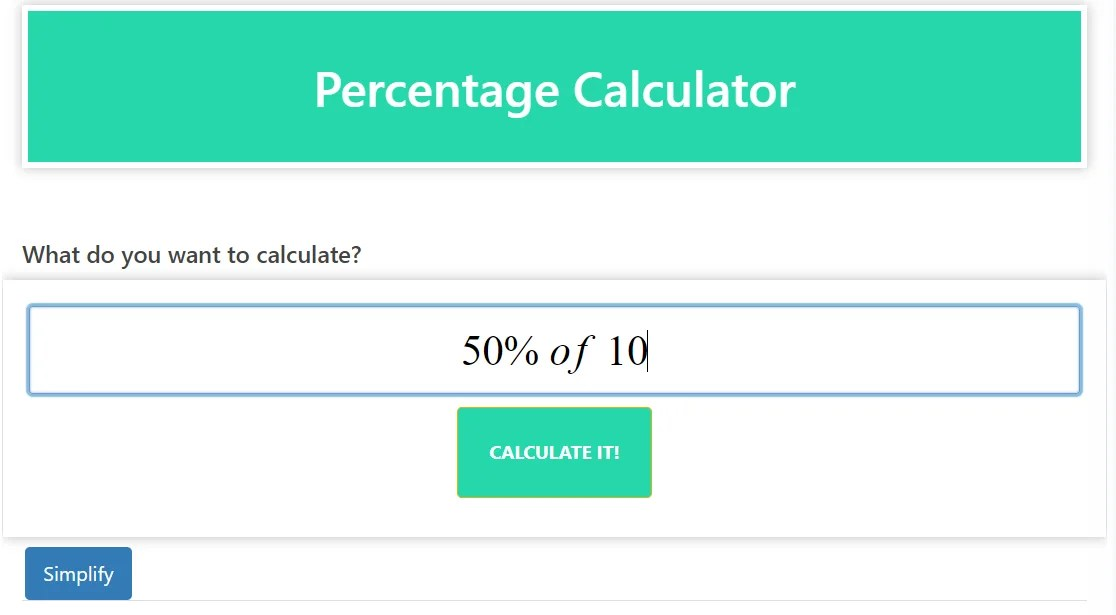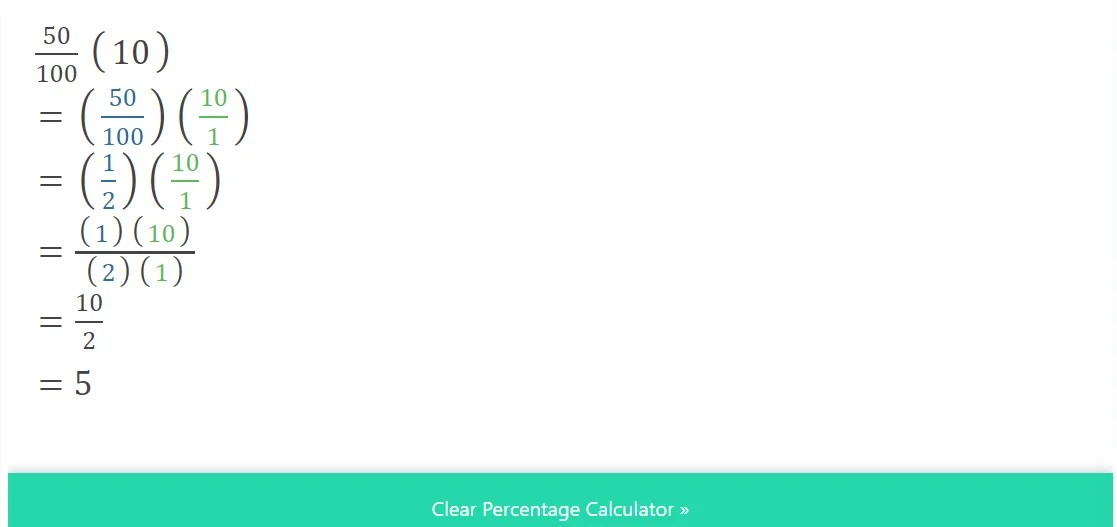# Percentage Calculator – Your Handy Math Solver

### Introduction:

Are you struggling with percentage calculations? look no further! Our percentage calculator is here to make solving your math problems easier. This versatile maths solver enables you to calculate percentages effortlessly while simplifying complex calculations.

### Explanation:

The percentage Calculator is a valuable tool for solving various percentage-related calculations. Percent is commonly used to express ratios, rates of change, and comparisons in multiple fields such as finance, statistics, and everyday life.

Our calculator provides a wide range of functionality, so you can efficiently perform a variety of percentage calculations. Here are some key features and use cases:

### Percentage Increase/Decrease:

With our Percentage Calculator, you can determine the percentage increase or decrease between two values. Whether you’re analyzing price change, population growth, or test scores, this calculator gives you accurate percentage changes.

### Percentage of a Whole:

Calculating the percentage of a given value with respect to the whole is a common task. Our calculator streamlines this process, so you can easily find the percentage of a number in a few simple steps.

### Percentage Difference:

It is necessary to compare two values in different scenarios and calculate the percentage difference. Our calculator efficiently determines the percentage difference between two numbers, making it ideal for analyzing data sets or assessing changes over time.

### Percentage Ratio:

Working with ratios and proportions often involves percentages. Our calculator enables you to solve percentage-based ratios quickly and accurately, helping you solve problems involving ratios, fractions, and comparisons.

Using our percentage calculator is straightforward. Simply input the required value or choose the appropriate calculation type, and the calculator will instantly provide you with the desired result. It is a time-saving tool that minimizes the chance of errors, allowing you to focus on analyzing and interpreting the data. Percentage Calculator (Maths solver)

### conclusion:

Simplify your percentage calculations and save time with our versatile Percentage Calculator. Whether you need to calculate percentage changes, find percentages of wholes, determine percentage differences, or solve percentage-based ratios, our math solver has you covered. Experience the convenience and accuracy of our percentage calculator today, and solve percentage calculations with ease. Percentage Calculator (Maths solver)